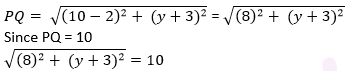Newbie

# Find the values of y for which the distance between the points P (2, – 3) and Q (10, y) is 10 units. Q.8

• 0

How i solve the  coordinate geometry questions easily . What is the best solution of  exercise 7.1  , please  help me to solve this question easily. Find the values of y for which the distance between the points P (2, – 3) and Q (10, y) is 10 units.

Share

1. Given: Distance between (2, – 3) and (10, y) is 10.

Using distance formula,Simplify the above equation and find the value of y.

Squaring both sides,

64 + (y + 3)2 = 100

(y + 3)= 36

y + 3 = ±6

y + 3 = +6 or y + 3 = −6

Therefore, y = 3 or -9.

• 0